'/declinhu.htm')) ?>

# To find the declination of a wall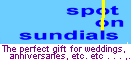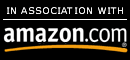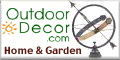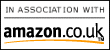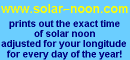The declination of a wall is the number of degrees east or west of south made by a perpendicular from the wall' surface. (In the southern hemisphere, it's the number of degrees east or west of north

(graphics to come)

Both figures above show a wall, CD, that declines from due south. In both cases the line OB is perpendicular to the wall and OA represents the meridian, with A to the south. The Angle of Declination is the angle AOB.

### METHOD 1:

Place a horizontal shelf against the wall (straight edge to the the wall, shelf level and perpendicular to the wall). Hold up a plumb line and when the sun souths, mark the position of the noon shadow. Since at the moment of local apparent noon the sun is due south, the shadow of

the plumb will lie in the plane of the meridian. If we mark its position

on the shelf it will give us the line OA. Draw a line perpendicular to the straight edge of the shelf intersecting OA at O, and measure the angle formed to determine the declination of the wall from true south.

### METHOD 2:

To find the declination of the wall at any time of the day, you need to determine: (a) the direction of the sun from the wall, and (b) the direction of the sun from the south point.
First determine the angle of the sun relative to the wall. This can be done by using a simple instrument constructed as shown in the figure. Tack a piece of paper to a flat board about a foot square, drive a nail (EF) into the board perpendicular to the face with about 3" left above the surface. Draw a straight line through the base of the nail (EW). Hang a weight by a thin thread at the base of the nail (E). To use, place the board against the wall and rotate till the plump line coincides with the line EW. Note the time for later use. Mark carefully the end of the shadow cast by the nail (C). Measure the perpendicular distance (CD) to the vertical line (EW). We now have two linear measurements, EF (the length of the nail above the surface of the board), and CD (the displacement of the end of the shadow from the vertical). Divide CD by EF, and the quotient is the tangent of the angle of the sun from the wall.

This gives us the first of our unknowns- the direction of the sun relative to the wall. We still need to know the direction of the sun from the south point of the horizon. This direction is called the sun's "azimuth from the south". This can be found for any combination of latitude, solar declination, and local apparent time in the proper published tables (Tables of Computed Altitude and Azimuth, U.S. Hydrographic Publication No. 214). Each volume covers 10 degrees of latitude with azimuth from the north. Once you have the right volume, subtract the listed azimuth from 180 degrees to yield results from the south.

Lacking tables, we compute the sun's azimuth, starting with the clock time (Mean Time) of our observation. Depending on the longitude of the observation, add or subtract from the standard meridian (90 degrees west in the central time zone) to obtain Local Mean Time, then add or subtract the proper amount from the Equation of Time for the day of the observation to obtain the Local Apparent Time. Convert the Local Apparent Time to an hour angle from noon (1hr =15 degrees, thus 9:36 A.M. being 2 hr. 24 min. before noon gives an hour angleof 36 degrees 0 min. 0 sec.). This value will become 't' in our computations.

Next we must know the sun's declination at the time of our observation. This can be taken from a current ephemeris (Ephemeris of the Sun, Polaris, and Other Selected Stars, Nautical Almanac Office of the U.S. Naval Observatory), or from one of the current almanacs. This will be value 'd'.

Finally, we must know the latitude of the place of our observation, 'theta'.

We proceed to find the sun?s azimuth by using these three values in solving two equations:

(1) tan M = tan d / cos t

(2) tan Z = [( tan t ) ( cos M )] / sin ( theta - M)

The intermediate value, M, is used to solve for Z, the sun?s azimuth. This is our second unknown.

In the figure*, AB is the wall, angle SOP = a = declination of the wall, POL = b = direction of the sun from the wall, SOL = c = direction of the sun from the south point (azimuth). Then:

#### (A) If the observation was 'before' local apparent noon:

(1) If the sun was to the right of the wall:

(a) The wall declines East by the amount c+b.

(2) If the sun was to the left of the wall:

(a) If b>c, the wall declines West by b-c.
(b) If c>b, the wall declines East by c-b.

#### (B) If the observation was 'after' local apparent noon:

(1) If the sun was to the left of the wall:

(a) The wall declines West by the amount c+b.

(2) If the sun was to the right of the wall:

(a) If b>c, the wall declines East by b-c.
(b) If c>b, the wall declines West by c-b.
Remember that in the winter the sun's declination is negative, so in solving the formulas, 'd' and M will be negative, and in getting the value of (theta - M) we subtract algebraically.

* refers to the example used in the figure.

REFERENCES: see our Books page

Sundials: How to Know, Use, and Make Them by R. Newton Mayall & Margaret W. Mayall

Sundials: Their Theory and Construction by Albert E. Waugh